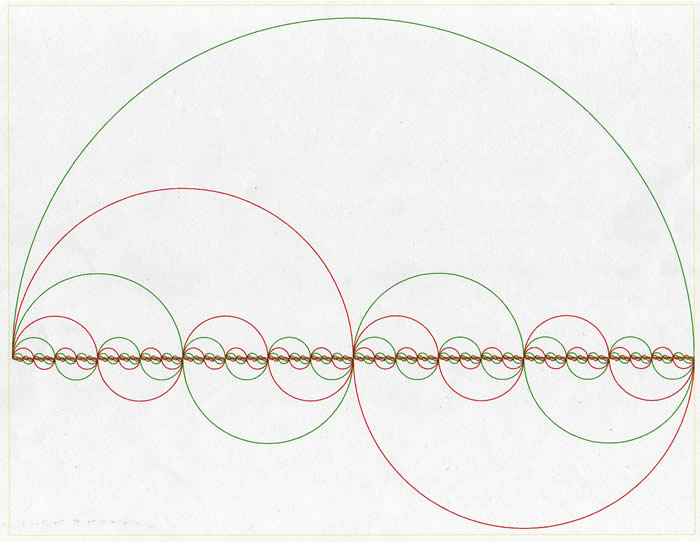# Dirk Bertels

• Music, Maths, Science and GIC (General Idle Contemplation)
The greatest malfunction of spirit
is to believe things (Louis Pasteur)

# PI ( Π ) = 3.1415 9265 3589 7932 3846 2643 3...

### Irrationality of PI

A great way to visualise the irrationality of PI is to think of a circle and its diameter. Pick a point on this circle, pick up the diameter, line up its starting point with this point and wrap it around the circle. Mark the endpoint of the wrapped diameter on the circle. Pick up the diameter again and line up its starting point with this last point, wrap around the circle again and create a new mark at the diameter's endpoint on the circle. Keep on doing this at infinitum. No matter how long you will do this - NO POINTS WILL EVER OVERLAP. If they did at some point, the length of the diameter would be a ratio of the circumference of the circle. PI being what it is, can never be expressed in the form of a ratio, x/y. Hence PI is irrational.

The above mental picture can be viewed upon as a geometric proof that PI has to be irrational. That is, the above procedure has to be ongoing since we need to derive all points of the circle which consists of an infinite amount of points.

### Paradox of the circle's circumference becoming its diameter

The idea used here is that the circumference of a circle is the same as the sum of the circumferences of 2 smaller circles that fit inside this circle.

Looking at the figure below, the length of the large green semi-circle above the midline equals the sum of the lengths of the largest red semi-circle above the midline and the largest red semi-circle below the midline. The shape thus formed resembles the 'tear' shape so characteristic of the yin-yang symbol.

In turn, the length of the largest red semi-circle above the midline equals the sum of the lengths of the 2 green semi-circles with which it forms a tear-drop.

Taking this idea further - thinking recursively - the length of the large green semi-circle on top of the midline is the same as the length of the tiny red wavy line squigling around the midline.

Knowing that PI represents the relationsip between a circle and its midline, the interaction of the 2 fundamental elements (circle and midline) is well illustrated here.

It is interesting to visualise this process going on at infinitum; the little red wavy line getting smaller and smaller as its frequency increases. Of course, we know that no matter how small this wavy line gets, its length will always be PI/2 times greater than the true midline (the division by 2 is because we are using half circles here).

Note also the binary aspect of this procedure whereby the large circle contains 2 smaller circles, which in turn contain 2 circles, etc.figure 1: cirumference - diameter paradox

### Program to calculate PI

Here is a recursive algorithm for calculating PI. It only needs 15 loops (recursions) to calculate PI exactly to 12 digits following the decimal point.

``````
// set initial values
a = x = 1;
b = 1 / sqrt(2);
c = 1 / 4;

// Recursive loop
// In reality, the loop needs to be bounded. Accuracy limited to
// the computer's limitations re length of type used and floating
// point processing capabilities.

for(;;)
{
y = a;
a = (a + b) / 2;
b = sqrt(by);
c = c - x(a - y)^2;
x = 2x;

print((a + b)^2 / 4c);
}

// first returned values are
// loop 1: 2.9142135
// loop 2: 3.1405797
// loop 3: 3.1415928
``````

### PI facts

Π (PI) is Greek for the letter P for Perimeter (of a circle).

After calculating PI to 16 million digits, it still passed all tests for randomness.